# JP4322548B2 - Data format conversion circuit - Google Patents

## Info

Publication number
JP4322548B2
JP4322548B2 JP2003132078A JP2003132078A JP4322548B2 JP 4322548 B2 JP4322548 B2 JP 4322548B2 JP 2003132078 A JP2003132078 A JP 2003132078A JP 2003132078 A JP2003132078 A JP 2003132078A JP 4322548 B2 JP4322548 B2 JP 4322548B2
Authority
JP
Japan
Prior art keywords
data
parallel
serial
means
output
Prior art date
Legal status (The legal status is an assumption and is not a legal conclusion. Google has not performed a legal analysis and makes no representation as to the accuracy of the status listed.)
Active
Application number
JP2003132078A
Other languages
Japanese (ja)
Other versions
JP2004336558A (en
Inventor

Original Assignee
Ｎｅｃエレクトロニクス株式会社

Priority date (The priority date is an assumption and is not a legal conclusion. Google has not performed a legal analysis and makes no representation as to the accuracy of the date listed.)
Filing date
Publication date
Application filed by Ｎｅｃエレクトロニクス株式会社, 日本電気株式会社 filed Critical Ｎｅｃエレクトロニクス株式会社
Priority to JP2003132078A priority Critical patent/JP4322548B2/en
Publication of JP2004336558A publication Critical patent/JP2004336558A/en
Application granted granted Critical
Publication of JP4322548B2 publication Critical patent/JP4322548B2/en
Application status is Active legal-status Critical
Anticipated expiration legal-status Critical

## Images

•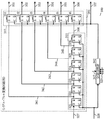•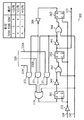•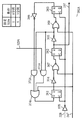••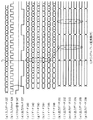•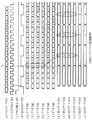•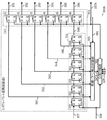•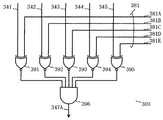•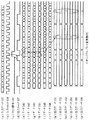•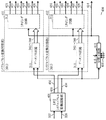•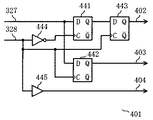•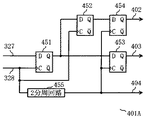•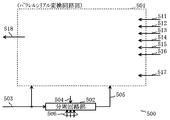•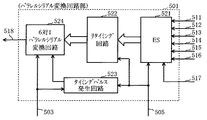•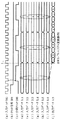•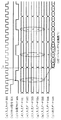•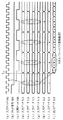••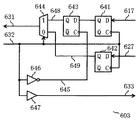•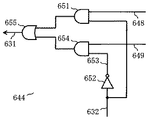•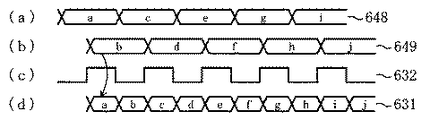••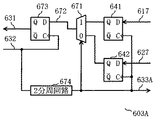•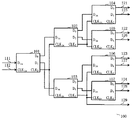•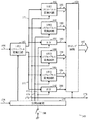•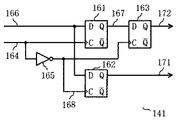•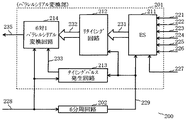## Classifications

• HELECTRICITY
• H03BASIC ELECTRONIC CIRCUITRY
• H03MCODING; DECODING; CODE CONVERSION IN GENERAL
• H03M9/00Parallel/series conversion or vice versa

## Description


BACKGROUND OF THE INVENTION
The present invention relates to a data format conversion circuit for converting parallel data or serial data, and more particularly to a data format conversion circuit for converting parallel data to serial data and vice versa.

[Prior art]
The progress of LSI (Large Scale Integration) technology is remarkable, the operating clock inside LSI is several hundred MHz (megahertz), and the signal transmission speed between LSIs is several Gbps (gigabit / second) class, Speeding up. On the other hand, the difference between the operation speed inside the LSI and the signal transmission speed between the LSIs is large. Therefore, if a parallel-serial conversion circuit is arranged in the output unit of the LSI and low-speed parallel data in the LSI is converted into high-speed serial data, the high-speed serial data can be output from the LSI to the outside. If a serial / parallel conversion circuit is arranged in the input section of the LSI, it is possible to convert high-speed serial data input from the outside into low-speed parallel data and supply it to the LSI.

For this purpose, several serial-parallel conversion circuits for converting serial data into parallel data and parallel-serial conversion circuits for converting parallel data into serial data have been proposed to meet the demand for data input / output between LSI and the outside. (For example, Patent Document 1, Patent Document 2, and Patent Document 3).

FIG. 24 shows the configuration of the first proposed serial-parallel conversion circuit disclosed in Patent Document 1. In FIG. This serial-parallel conversion circuit 100 connects first to seventh 1-to-2 DEMUX (De-Multiplexer) modules 101 to 107 having the same structure so as to form a tree-like multistage structure. Each of the first to seventh one-to-two DEMUX modules 101 to 107 is a basic module that converts one signal into a plurality of lower-speed digital signals and outputs them. These basic modules have a data input terminal DinAnd clock input terminal CLKinAre provided on the input side, and the first and second data output terminals D are provided on the output side.0, D1And clock output terminal CLK2It has.

Data input terminal D of first one-to-two DEMUX module 101inIs supplied with input data 111, and clock input terminal CLKinIs supplied with an input clock 112. Its first data output terminal D0Is the data input terminal D of the second one-to-two DEMUX module 102 in the second stage.inTo the second data output terminal D1Is the data input terminal D of the third one-to-two DEMUX module 103 in the second stage.inTo be connected to. Clock output terminal CLK of the first one-to-two DEMUX module 1012Are the clock input terminals CLK of the second and third one-to-two DEMUX modules 102, 103.inIt is connected to the. The same applies hereinafter, the output side of the second one-to-two DEMUX module 102 is connected to the input side of the fourth and fifth one-to-two DEMUX modules 104 and 105, and the output side of the third one-to-two DEMUX module 103 is the first 6 and the seventh one-to-two DEMUX modules 106 and 107 are connected to the input side.

Next, the operation of the serial / parallel conversion circuit 100 shown in FIG. 24 will be described. Input data 111 is high-speed serial input data. The input clock 112 is a high-speed clock synchronized with this. The first one-to-two DEMUX module 101 converts the input data 111 into two parallel data at a half rate, and the first or second data output terminal D0, D1Are output to the corresponding ones. At this time, the clock output terminal CLK of the first one-to-two DEMUX module 1012Outputs a clock having a half rate of the input clock 112.

Similarly, the second one-to-two DEMUX module 102 and the third one-to-two DEMUX module 103 in the second stage similarly output the data and clock output from the first one-to-two DEMUX module 101 at two half rates. Convert to parallel data and half rate clock. Similarly, the fourth to seventh one-to-two DEMUX modules 104 to 107 in the third stage also convert the input data and clock into a clock with a half rate as well as two parallel data at a half rate. Convert. The final data 121 to 128 converted in this way is data obtained by converting the high-speed serial input data 111 into low-speed parallel data at a ratio of 1: 8. The output clock 129 is a clock synchronized with the final data 121-128.

FIG. 25 shows the configuration of the second proposed serial-parallel conversion circuit disclosed in Patent Document 2. In FIG. The serial / parallel conversion circuit 140 includes a first one-to-two serial / parallel conversion circuit 141 disposed in the first stage and second to fifth one-to-two serial / parallel conversion circuits disposed in the second stage. 142 to 145, first and second flip-flop circuits (F / F) 151 and 152 arranged in the second stage, and these second to fifth one-to-two serial / parallel conversion circuits 142 to 145, , A retiming circuit 153 arranged on the output side of the first and second flip-flop circuits 151 and 152, and a frequency dividing circuit unit 155 that divides the input clock 154 and a predetermined terminal of the frequency dividing circuit unit 155 and the ground And an on / off switch 156 disposed therebetween.

FIG. 26 shows a specific circuit configuration of the first one-to-two serial / parallel conversion circuit shown in FIG. Since the second to fifth one-to-two serial / parallel conversion circuits 142 to 145 have the same circuit configuration as that of the first one-to-two serial / parallel conversion circuit 141, illustration and description of these circuits are omitted. The first one-to-two serial / parallel conversion circuit 141 includes first to third flip-flop circuits 161 to 163 and an inverter circuit 165 that inverts an input clock 164. The input data 166 is input to the input terminals D of the first and second flip-flop circuits 161 and 162, and the output 167 of the output terminal Q of the first flip-flop circuit 161 is input to the input terminal D of the third flip-flop circuit 163. To be input. The input clock 164 is input to the clock input terminal C of the first flip-flop circuit 161. The clock 168 whose logic is inverted by the inverter circuit 165 is input to the clock input terminal C of the second and third flip-flop circuits 162 and 163. In the first one-to-two serial / parallel conversion circuit 141, the two parallel data 171 and 172 are output from the second and third flip-flop circuits 162 and 163.

Returning to FIG. 25, the operation of the serial-parallel conversion circuit 140 will be described. The high-speed serial input data 166 is input to the first one-to-two serial / parallel conversion circuit 141 and converted into two parallel data 171 and 172 (see FIG. 26) at a half rate. One of the parallel data 171 is input to the second and third one-to-two serial / parallel conversion circuits 142 and 143 and the first flip-flop circuit 151. The other parallel data 172 is input to the fourth and fifth one-to-two serial / parallel conversion circuits 144 and 145 and the second flip-flop circuit 152. Here, the operation clock 173 of the second and fourth one-to-two serial / parallel conversion circuits 142 and 144 is obtained by dividing the input clock 154 by the frequency dividing circuit 155. The operation clock 174 of the third and fifth one-to-two serial / parallel conversion circuits 143 and 145 is obtained by dividing the input clock 154 by the frequency dividing circuit 155. The operation clock 175 of the first and second flip-flop circuits 151 and 152 is a clock obtained by dividing the input clock 154 by the frequency dividing circuit 155.

The operation clocks 173 to 175 output from the frequency dividing circuit 155 have the same frequency but different phases. An on / off switch 156 is connected to the frequency dividing circuit 155. The on / off switch 156 divides the input clock 154 by two types of frequency division ratios with respect to the frequency dividing circuit 155 by setting it to an on state or an off state, and the operation clocks 173 to 175 are divided. Can be output. The output data 181 to 186 of the second to fifth one-to-two serial / parallel conversion circuits 142 to 145 and the first and second flip-flop circuits 151 and 152 are retimed by the operation clock 173 in the retiming circuit 153. . As a result, parallel data 187 is output. The operation clock 173 used for retiming is output as an output clock. This operation clock 173 is synchronized with the parallel data 187.

FIG. 27 shows a configuration of a parallel-serial conversion circuit as a third proposal disclosed in Patent Document 3. The parallel-serial conversion circuit 200 performs 6-to-1 parallel-serial conversion, and includes a parallel-serial conversion unit 201 and a 6-frequency divider circuit 202. The parallel-serial conversion unit 201 converts an elastic store circuit (ES) 211, a retiming circuit 212 that performs retiming, a timing pulse generation circuit 213 that generates timing pulses, and parallel data after retiming into serial data. 6 to 1 parallel-serial conversion circuit 214.

The operation of the parallel-serial conversion circuit 200 will be described. First to sixth input data 221 to 226 are supplied as parallel input data to the elastic store circuit 211, and writing to the elastic store circuit 211 is performed in synchronization with the write clock 227. The parallel data written in the elastic store circuit 211 is read by the read clock 229 obtained by dividing the input clock 228 by 6 by the 6-frequency divider circuit 202. The read parallel data 231 is retimed by the retiming circuit 212. The retimed parallel data 232 is converted into serial output data 235 by the 6-to-1 parallel-serial conversion circuit 214 based on the timing pulse 233 generated by the timing pulse generation circuit 213 and output.

[Patent Document 1]
JP-A-11-98101 (paragraphs 0016 and 0017, FIGS. 4 and 5)
[Patent Document 2]
JP 2002-217742 A (paragraphs 0013 to 0015, FIG. 1)
[Patent Document 3]
JP-A-8-65173 (paragraphs 0016 to 0019, FIG. 1)

[Problems to be solved by the invention]
However, in the first proposed serial / parallel conversion circuit 100 shown in FIG. 24, the one-to-two DEMUX modules 101 to 107 represented by the first one-to-two DEMUX module 101 are connected in multiple stages in a tree shape. The serial data is converted into parallel data at specific ratios of 1: 2, 1: 4, 1: 8 or 1:16 at each stage of the tree structure. Therefore, while serial / parallel conversion can be performed at a ratio that is a factorial of 2 in this way, there is a problem that serial data cannot be converted into parallel data at other ratios.

The third proposal shown in FIG. 27 also uses a frequency dividing circuit that divides the frequency by a predetermined frequency dividing ratio. For this reason, there is a problem that the number of low-speed parallel data to be converted into serial data is determined in one way depending on the number of parallel data input by the frequency dividing circuit. As a result, for example, when performing parallel-serial conversion at two different ratios of 4 to 1 and 5 to 1, it is necessary to design a total of two types of parallel-serial conversion circuits individually corresponding to each ratio. There is a problem that the design amount increases.

On the other hand, in the second proposal shown in FIG. 25 and FIG. 26, two types of frequency division ratios can be set by setting the on / off switch 156 to the on or off state. Moreover, by designing one circuit, there is an advantage that serial / parallel conversion can be performed with two kinds of ratios as the number of states of the on / off switch 156. However, in the second proposal, a plurality of divided clocks are generated from the frequency dividing circuit 155. Therefore, attention must be paid to the skew between the divided clocks. For example, there is a possibility that the setup and holding (hold time) of the first and second flip-flop circuits 151 and 152 may become strict at locations where data transfer occurs between the divided clocks. Therefore, it is necessary to confirm that there is enough time for these times. Therefore, there is a problem that the difficulty of design becomes difficult by this amount.

SUMMARY OF THE INVENTION Accordingly, an object of the present invention is to provide a data format conversion circuit capable of performing parallel-serial conversion or serial-parallel conversion at various ratios.

[Means for Solving the Problems]
In the present invention, (a) an input clock synchronized with serial data to be converted is set in a plurality of ways according to predetermined division ratio setting information, divided, and output as an output clock on one signal line. (B) a frequency division ratio setting means for setting the frequency division ratio of the frequency division means to any one of a plurality of predetermined numbers using the frequency division ratio setting information described above; )A data shift means for sequentially shifting the serial data described above at the timing of the input clock; and a retiming means for retiming the output data of the data shift means by the clock divided by the frequency dividing means,The serial data to be convertedAs described aboveSerial / parallel conversion means for converting into parallel data having the number of bits corresponding to the division ratio set by the division ratio setting means;Synchronization pattern detection means for comparing the output data of the data shift means before retiming by the retiming means and a synchronization pattern set externally;)Resetting means for resetting the frequency dividing means at the timing when the synchronous pattern detecting means detects the synchronous pattern and starting the frequency division of the input clock;The parallel data after conversion by the serial / parallel conversion meansAs described aboveThe data format conversion circuit comprises parallel data output means for outputting in synchronization with the output clock of the frequency dividing means.

In the present invention, (a) a first-stage serial / parallel conversion unit that converts input serial data into parallel data having a predetermined number of bits in synchronization with a predetermined input clock; and (b) conversion by the first-stage serial / parallel conversion unit. 1 is prepared for each serial data consisting of a series of 1-bit data constituting each parallel data, and the input clock described above is set in a plurality of ways according to predetermined division ratio setting information, and is divided. Frequency dividing means capable of outputting as an output clock to one signal line, and a frequency dividing ratio of the frequency dividing means is set to any one of a plurality of predetermined methods using the frequency division ratio setting information described above. Frequency division ratio setting means, data shift means for sequentially shifting the serial data at the timing of the input clock, and output data of the data shift means Retiming means for retiming with a clock frequency-divided by the frequency dividing means described above, and paralleling the number of bits corresponding to the frequency dividing ratio set by the frequency dividing ratio setting means described above Next-stage serial / parallel conversion means for converting to data, synchronization pattern detection means for comparing the output data of the data shift means before retiming by the retiming means and a synchronization pattern set from the outside, and this synchronization The reset means for resetting the frequency dividing means at the timing when the pattern detecting means detects the synchronization pattern and starting the frequency division of the input clock, and the parallel data after the conversion of the next-stage serial / parallel converting means Parallel data output means for outputting in synchronization with the aforementioned output clock of the frequency dividing means The data format conversion circuit includes a next-stage serial / parallel conversion unit including the parallel data output from each of the subsequent-stage serial / parallel conversion units, and the parallel data is synchronized with the output clock. .

Furthermore, in the present invention, (b) an output synchronous clock synchronized with the output serial data can be set in a plurality of ways according to predetermined division ratio setting information and divided to be output as an output clock to one signal line. A frequency dividing means, a frequency dividing ratio setting means for setting a frequency dividing ratio of the frequency dividing means to any one of a plurality of predetermined values using the frequency dividing ratio setting information, and the frequency dividing means. Parallel data fetching means for fetching parallel data of the number of bits corresponding to the frequency division ratio set by the ratio setting means, and the parallel data fetched by the parallel data fetching means are output by the output clock output from the frequency dividing means. Retiming means for timing, and parallel data retimed by the retiming means is converted into serial data in synchronization with the output synchronous clock and output. A plurality of parallel serial conversion units having parallel serial conversion means, and (b) a next-stage parallel serial conversion unit that inputs serial data output from each of these parallel serial conversion units in parallel and outputs the data as serial data. A format conversion circuit is provided.

Furthermore, in the present invention, (b) an output synchronous clock synchronized with the output serial data is set in a plurality of ways in accordance with predetermined division ratio setting information, and is divided and output to one signal line as an output clock. Possible frequency dividing means, frequency dividing ratio setting means for setting the frequency dividing ratio of the frequency dividing means to any one of a plurality of predetermined values using the above-described frequency dividing ratio setting information, Parallel data fetching means for fetching parallel data of the number of bits corresponding to the frequency division ratio set by the frequency ratio setting means, and the parallel data fetched by the parallel data fetching means are output clocks output from the frequency dividing means. Retiming means for retiming, and parallel data retimed by the retiming means are converted into serial data in synchronization with the output synchronous clock and output. Parallel serial conversion means, and a plurality of parallel serial conversion units arranged in a tree structure, and (b) two or more of the plurality of parallel serial conversion units positioned at the apex side end of the tree structure described above The data format conversion circuit includes a vertex parallel serial conversion unit that inputs serial data output from the parallel serial conversion unit in parallel and outputs the serial data as serial data.

DETAILED DESCRIPTION OF THE INVENTION

【Example】
Hereinafter, the present invention will be described in detail with reference to examples.

<First embodiment>

FIG. 1 shows a serial-parallel conversion circuit as a first embodiment of the data format conversion circuit of the present invention. The serial / parallel conversion circuit 300 includes a serial / parallel conversion circuit unit 301 and a frequency dividing circuit unit 302. In the serial / parallel conversion circuit 300 according to the first embodiment, the serial / parallel conversion circuit unit 301 includes a data shift circuit 311 and a retiming circuit 312. The data shift circuit 311 has a structure in which the first to sixth flip-flop circuits 321 to 326 are connected in cascade, and supplies the input data 327 to the data data input terminal D of the first flip-flop circuit 321. Are sequentially shifted by the input clock 328. The input clock 328 is also supplied to the frequency dividing circuit 302 so that various frequency division ratios can be set by a frequency division ratio setting signal 329.

Similarly, the retiming circuit 312 includes first to sixth flip-flop circuits 331 to 336, and an output clock 337 output from the frequency divider circuit unit 302 is input to the clock input terminal C thereof. The first flip-flop circuit 331 of the retiming circuit 312 supplies the data input terminal D of the second flip-flop circuit 322 of the next stage from the output terminal Q of the first flip-flop circuit 321 of the data shift circuit 311. Data 341 to be input is input to the data input terminal D. The second flip-flop circuit 332 supplies data 342 supplied from the output terminal Q of the second flip-flop circuit 322 of the data shift circuit 311 to the data input terminal D of the third flip-flop circuit 323 of the next stage. The data is input to the data input terminal D. The same applies hereinafter. In the case of the sixth flip-flop circuit 336, the data 346 output from the output terminal Q of the sixth flip-flop circuit 326 of the data shift circuit 311 is input to the data input terminal D.

In the serial / parallel conversion circuit 300, serial input data 327 input from the outside and an input clock 328 synchronized therewith are supplied to the serial / parallel conversion circuit unit 301. The input data 327 sequentially shifts the first to sixth flip-flop circuits 321 to 326 in the data shift circuit 311. The input clock 328 is also input to the frequency dividing circuit unit 302. The frequency divider circuit 302 is reset by the reset signal 347, divides the input clock 328 by a frequency division ratio corresponding to the frequency division ratio setting signal 329, and outputs the frequency-divided output clock 337. . In the first to sixth flip-flop circuits 331 to 336 in the retiming circuit 312, the data 341 to 346 output from the flip-flop circuits 321 to 326 of each stage of the data shift circuit 311 are received at the rising edge of the output clock 337. Retiming is performed, and output data 351 to 356 are output from the output terminals Q of the first to sixth flip-flop circuits 331 to 336 of the retiming circuit 312.

FIG. 2 shows a specific configuration of the frequency dividing circuit section shown in FIG. The frequency divider 302 includes first to third flip-flop circuits 361 to 363, first to third inverters 364 to 366 connected to the output terminals Q, and first and second inverters. NOR circuits 367 and 368 in which one input side is connected to the output side of each of the inverters 364 and 365, and first to third connected to the output side of the second inverter 365 or the third inverter 366. AND circuits 371 to 373, and an OR circuit in which the input side is arranged on the output side of these AND circuits 371 to 373 and the output side is connected to the input terminal D of the first flip-flop circuit 361. 374 and a fourth inverter 375 that inputs the inverted clock of the input clock 328 to the clock input terminal C of the first flip-flop circuit 361. It is.

The frequency divider circuit 302 divides the input clock 328 in synchronization with the falling edge of the input clock 328, and there are three frequency dividing ratios corresponding to the setting of the frequency dividing ratio setting signals 329A to 329C. To change. That is, when the frequency division ratio setting signal 329A is set to “1”, the frequency division ratio setting signal 329B is set to “0”, and the frequency division ratio setting signal 329C is set to “0”, the circuit is divided by four.

Further, when the frequency division ratio setting signal 329A is set to “0”, the frequency division ratio setting signal 329B is set to “1”, and the frequency division ratio setting signal 329C is set to “0”, the circuit becomes a frequency dividing circuit. If the frequency division ratio setting signal 329A is set to “0”, the frequency division ratio setting signal 329B is set to “0”, and the frequency division ratio setting signal 329C is set to “1”, a 6 frequency dividing circuit is obtained.

FIG. 3 shows another example of the frequency dividing circuit shown in FIG. The same parts as those in FIG. 2 are denoted by the same reference numerals, and description thereof will be omitted as appropriate. In the frequency dividing circuit section 302A, the output of the second inverter 365 is input to one input terminal of the two-input first and second AND circuits 371A and 372A. A frequency division ratio setting signal 329A (see FIG. 2) is input to the other input terminal of the first AND circuit 371A. An output after the inversion of the third inverter 366 is supplied to the other input terminal of the second AND circuit 372A. The OR circuit 374A calculates the logical sum of the outputs of the first and second AND circuits 371A and 372A and supplies the output to the data input terminal D of the first flip-flop circuit 361.

In such a frequency dividing circuit unit 302A, the frequency dividing ratio changes in two ways according to the setting of the frequency dividing ratio setting signal 329A. That is, if the frequency division ratio setting signal 329A is set to “1”, the circuit becomes a frequency division circuit. If the frequency division ratio setting signal 329A is set to “0”, a 5 frequency dividing circuit is formed.

FIG. 4 shows various timings when a 1 to 6 serial-parallel conversion operation is performed with a frequency dividing ratio of 6. This is a case where the frequency dividing circuit unit 302 shown in FIG. 2 is used. On the other hand, FIG. 5 shows a case where a 1 to 5 serial-parallel conversion operation is performed with a frequency dividing ratio of 5. Further, FIG. 6 shows a case where a serial-to-parallel conversion operation of 1 to 4 is performed with a frequency dividing ratio of 4. 5 and 6 can use both the frequency dividing circuit portion 302 shown in FIG. 2 and the frequency dividing circuit portion 302A shown in FIG.

When the reset signal 347 shown in FIG. 1 is changed from the signal “1” to the signal “0” at a predetermined time (FIG. 4C, FIG. 5C, FIG. 6C), it is shown in FIG. The frequency divider circuit 302 or the frequency divider circuit 302A shown in FIG. 3 (hereinafter, the frequency divider circuit 302 shown in FIG. 2 will be described for the sake of simplicity, and the frequency divider shown in FIG. The description of the circuit portion 302 is omitted as appropriate. The frequency division of the input clock 328 (FIGS. 4B, 5B, and 6B) is started. As a result, the output clock 337 (FIG. 4D, FIG. 5D, and FIG. 6D) rises at the first fall of the input clock 328. Hereinafter, as shown in FIGS. 4 to 6, as the output clock 337, the clock of 6 cycles of the input clock 328 is output in the case of FIG. 4, and the clock of 5 cycles of the input clock 328 in the case of FIG. In the case of FIG. 6, four cycles of the input clock 328 are output.

The overall operation of the serial / parallel conversion circuit 300 in the frequency dividing circuit 302 will be described with reference to FIGS. The input data 327 shown in FIGS. 4A, 5A, and 6A is shifted in accordance with the input clock 328 by the data shift circuit 311 shown in FIG. As a result, data 341 to 346 (FIGS. 4E to 4J and 5E to 5E) output from the output terminals Q of the first to sixth flip-flop circuits 321 to 326 (FIG. 1), respectively. (J) and FIGS. 6E to 6J are shifted by one clock at the rising timing of the input clock 328 (FIGS. 4A, 5A, and 6A). (See symbols a, b, c,... Attached to the input data 327.) Such data 341 to 346 are input to the retiming circuit 312 and retimed at the rising edge of the output clock 337. In this manner, output data 351 to 356 (FIGS. 4 (k) to (p), FIGS. 5 (k) to (p), and FIGS. 6 (k) to (p)) are output.

Here, in FIG. 4, the output data 351 to 356 are data obtained by serially converting the input data 327 to 1: 6. In FIG. 5, the output data 351 to 356 are serialized from the input data 327 to 1: 5. The data is converted into parallel data. In FIG. 6, output data 351 to 356 are data obtained by serial-to-parallel conversion of input data 327 on a one-to-four basis.

The case of dividing by 6 in FIG. 4 will be specifically described. For example, time t indicated by a broken line1Pay attention to. Assume that the input data 327 shown in FIG. 5A is composed of data “a”, “b”,. These are sequentially shifted by the first to sixth flip-flop circuits 321 to 326, and as a result, the time t1The data “f” is output from the first flip-flop circuit 321, and the data “e” is output from the second flip-flop circuit 322. Thereafter, the data “a” is output from the sixth flip-flop circuit 326 in the same manner. These data “f”, “e”,..., “A” are taken into the first to sixth flip-flop circuits 331 to 336 at the rising edge of the output clock 337 serving as a divided-by-6 clock. As a result, during one cycle of the output clock 337 until the next output clock 337 is output, data “f” is output as the output data 351 to 356 from the output terminals Q of the first to sixth flip-flop circuits 331 to 336. , “E”,..., “A” are output in parallel.

Also in the case of dividing by 5 in FIG. 5, for example, the time t shown by a broken line2Pay attention to. Assume that the input data 327 shown in FIG. 6A is composed of data “a”, “b”,. These are sequentially shifted by the first to fifth flip-flop circuits 321 to 325, and as a result, the time t2The data “e” is output from the first flip-flop circuit 321, and the data “d” is output from the second flip-flop circuit 322. Thereafter, data “a” is output from the fifth flip-flop circuit 325 in the same manner. These data “e”, “d”,..., “A” are taken into the first to fifth flip-flop circuits 331 to 335 at the rising edge of the output clock 337 as a divided-by-5 clock. As a result, during one cycle of the output clock 337 until the next output clock 337 is output, data “e” is output as the output data 351 to 355 from the output terminal Q of the first to fifth flip-flop circuits 331 to 335. , “D”,..., “A” are output in parallel.

Also in the case of the divide-by-four in FIG.ThreePay attention to. Assume that the input data 327 shown in FIG. 6A is composed of data “a”, “b”,. These are sequentially shifted by the first to fourth flip-flop circuits 321 to 324, and as a result, the time tThreeThe data “d” is output from the first flip-flop circuit 321, and the data “c” is output from the second flip-flop circuit 322. Thereafter, data “a” is output from the fourth flip-flop circuit 324 in the same manner. These data “d”, “c”,..., “A” are taken into the first to fourth flip-flop circuits 331 to 334 at the rising edge of the output clock 337 serving as a divided-by-4 clock. As a result, during one cycle of the output clock 337 until the next output clock 337 is output, data “d” is output from the output terminals Q of the first to fourth flip-flop circuits 331 to 334 as output data 351 to 354. , “C”,..., “A” are output in parallel.

As described above, the frequency dividing circuit 302 according to the present embodiment changes the frequency dividing ratio in response to the frequency dividing ratio setting signal 329 set from the outside. It can operate even when the number of side data is plural. Therefore, it is not necessary to design a serial-parallel conversion circuit according to each number, and the design amount can be greatly reduced.

Furthermore, in this embodiment, since it has a simple configuration that operates only with the input clock 327 and one frequency-divided clock 337, the design of the serial-parallel conversion circuit 300 becomes very easy.

<Modification of the first embodiment>

FIG. 7 shows a modification of the first embodiment. In FIG. 7, the same parts as those in FIG. The serial-parallel conversion circuit 300A of this modification has a configuration in which a synchronization pattern detection circuit unit 303 is disposed between the serial-parallel conversion circuit unit 301 shown in FIG.

The synchronization pattern detection circuit unit 303 includes data 341 to 346 output from the output terminals Q of the first to sixth flip-flop circuits 321 to 326 constituting the data shift circuit 311 and a synchronization pattern setting signal input from the outside. 381 is input. The synchronization pattern detection circuit unit 303 outputs a reset signal 347A when the data 341 to 346 and the synchronization pattern setting signal 381 match. As a result, the output clock 337 A is output from the frequency divider circuit 302 and supplied to the retiming circuit 312.

FIG. 8 shows a specific configuration of the synchronization pattern detection circuit unit. The synchronization pattern detection circuit unit 303 includes first to fifth exclusive-NOR (EX-NOR) circuits 391 to 395 and a 5-input AND circuit 396 arranged on the output side thereof. The first exclusive-NOR circuit 391 is supplied with the data 341 and the signal 381A of the first bit of the parallel data constituting the synchronization pattern setting signal 381. The second exclusive-nor circuit 392 is supplied with the data 342 and a signal 381B of the second bit of the parallel data constituting the synchronization pattern setting signal 381. Similarly, the fifth exclusive-NOR circuit 395 receives the data 345 and the signal 381E of the fifth bit of the parallel data constituting the synchronization pattern setting signal 381. The output of the 5-input AND circuit 396 becomes the reset signal 347A.

FIG. 9 shows the operation of each part of the serial-parallel conversion circuit shown in FIG. 9, the same parts as those in FIG. 5 are denoted by the same reference numerals, and description thereof will be omitted as appropriate. This figure shows the timing at the time of the 1 to 5 serial / parallel conversion operation in which the frequency division ratio of the serial / parallel conversion circuit 300A is "5". In this example, as shown in FIGS. 9E to 9H, when the data 341 to 344 (data “f” to “c”) match the corresponding signal patterns of the synchronization pattern setting signals 381A to 381D. As a result, the reset signal 347A becomes the signal “1”. Here, data “a”, “b”,... Are both binary signals of signal “1” or “0”.

That is, the synchronization pattern detection circuit unit 303 sets a 4-bit synchronization pattern composed of an arbitrary combination of the signal “1” and the signal “0” by the synchronization pattern setting signals 381A to 381D. Then, when the 4-bit data “f” to “c” matching the 4-bit synchronization pattern is shifted as the data 341 to 344 (FIGS. 9E to 9H) output from the data shift circuit 311. As shown in FIG. 9C, the reset signal 347A is set to the signal “1”. The frequency divider 302 is reset when the reset signal 347A becomes the signal “1”. As a result, the output clock 337A becomes the signal “0” at the falling timing of the input clock 328 (FIG. 9B), and becomes the signal “1” at the next falling timing. As a result of retiming the data 341 to 346 (FIGS. 9E to 9J) at the rising timing of the output clock 337A by the retiming circuit 312, the synchronization patterns “f” to “c” are aligned, and the output data Data “f” to “c” are output to 352 to 355, respectively. At this time, data “g” is output as the remaining output data 351. These output data 351 to 355 are the results of 1-to-5 serial / parallel conversion of the input data 327.

Thus, in this modification, the synchronization pattern can be aligned and output to the output data simply by using the reset signal 347A of the frequency dividing circuit 302 and adding the synchronization pattern detection circuit 303.

<Second embodiment>

FIG. 10 shows a serial-parallel conversion circuit according to the second embodiment of the present invention. In the serial / parallel conversion circuit 400 of the second embodiment, the input data 327 and the input clock 328 are first input to the one-to-two serial / parallel conversion circuit unit 401. The one-to-two serial / parallel conversion circuit unit 401 performs serial / parallel conversion on the input data 327 and outputs two parallel output data 402 and 403 and an output clock 404. Among these, the first parallel output data 402 is supplied to the first serial / parallel conversion circuit unit 411, and the second parallel output data 403 is supplied to the second serial / parallel conversion circuit unit 412. The output clock 404 is supplied to the first and second serial / parallel conversion circuit units 411 and 412 as a clock signal, and is also supplied to the frequency dividing circuit unit 413 as a clock signal.

The first and second serial / parallel conversion circuit units 411 and 412 have the same circuit configuration as the serial / parallel conversion circuit unit 301 shown in FIG. 1 of the first embodiment. The frequency divider 413 has the same circuit configuration as that of the frequency divider 302 in the first embodiment. That is, the divided first parallel output data 402 is input to the data shift circuit 311 in the first serial / parallel conversion circuit unit 411, and the first to sixth flip-flop circuits 331 shown in FIG. 341 to 336 are input to the retiming circuit 312, and retiming is performed at the rising edge of the output clock 414 output from the frequency divider 413 (corresponding to the output clock 337 of the first embodiment). To do. Then, output data 421 to 426 (corresponding to the output data 351 to 356 in FIG. 1) are output from the output terminals Q of the first to sixth flip-flop circuits 331 to 336 shown in FIG. It is like that.

Similarly, the second parallel output data 403 after frequency division is input to the data shift circuit 311 in the second serial / parallel conversion circuit unit 412, and the first to sixth flip-flop circuits 331 shown in FIG. 341 to 336 are input to the retiming circuit 312, and retiming is performed at the rising edge of the output clock 414 output from the frequency divider 413 (corresponding to the output clock 337 of the first embodiment). To do. Then, output data 431 to 436 (corresponding to the output data 351 to 356 in FIG. 1) are output from the output terminals Q of the first to sixth flip-flop circuits 331 to 336 shown in FIG. It is like that.

In the second embodiment, the frequency divider 413 changes the reset signal 415 corresponding to the reset signal 347 shown in FIG. 1 of the first embodiment from the signal “1” to the signal “0” (FIG. 4). (C), FIG. 5 (c), and FIG. 6 (c)), the frequency division of the output clock 404 is started. This corresponds to the start of frequency division of the input clock 328 (FIG. 4B, FIG. 5B, FIG. 6B) in the first embodiment. The frequency dividing circuit unit 413 can set various frequency dividing ratios by the frequency dividing ratio setting signal 416, which corresponds to setting various frequency dividing ratios by the frequency dividing ratio setting signal 329 in the embodiment shown in FIG. .

FIG. 11 shows a specific configuration of the one-to-two serial / parallel conversion circuit unit in the second embodiment. The one-to-two serial / parallel conversion circuit unit 401 includes first and second flip-flop circuits 441 and 442 that supply input data 327 to the data input terminal D, and an output terminal Q and a data input of the first flip-flop circuit 441. The circuit includes a third flip-flop circuit 443 connected to the terminal D, an inverter 444 that inverts the logic of the input clock 328, and a delay circuit 445 that gives a predetermined time delay. The output of the inverter 444 is supplied to the clock input terminal C of the first flip-flop circuit 441, and the input clock 328 is supplied as it is to the clock input terminal C of the second and third flip-flop circuits 442 and 443. In the one-to-two serial / parallel conversion circuit unit 401, one parallel output data 402 is output from the output terminal Q of the third flip-flop circuit 443, and the other parallel output is output from the output terminal Q of the second flip-flop circuit 442. Data 403 is output. Further, the output of the delay circuit 445 becomes the output clock 404.

In the one-to-two serial / parallel conversion circuit unit 401 shown in FIG. 11, the input data 327 is retimed at both rising and falling timings of the input clock 328. The frequency of the input clock 328 is half the frequency of the input data 327.

FIG. 12 shows another one-to-two serial / parallel conversion circuit applicable to the serial / parallel conversion circuit of FIG. The one-to-two serial / parallel conversion circuit unit 401A includes a first flip-flop circuit 451 that inputs input data 327 to the data input terminal D, an output terminal Q of the first flip-flop circuit 451, and a data input terminal D. The second and third flip-flop circuits 452 and 453 connected to each other, the fourth flip-flop circuit 454 connected to the output terminal Q of the second flip-flop circuit 452 and the data input terminal D, and the input clock 328 are input. Thus, it is constituted by a divide-by-2 circuit 455 that divides this frequency by two. The input clock 328 is also supplied to the clock input terminal C of the first and second flip-flop circuits 451 and 452. Further, the output clock 404 obtained by the frequency division by the frequency divider circuit 455 is supplied to the first and second serial / parallel conversion circuit units 411 and 412 of FIG. 10, and the third and fourth flip-flop circuits. The clock input terminals C 453 and 454 are also supplied.

The one-to-two serial / parallel conversion circuit unit 401A shown in FIG. 12 has a simple circuit configuration similar to the one-to-two serial / parallel conversion circuit unit 401 shown in FIG. In the one-to-two serial / parallel conversion circuit unit 401A in FIG. 12, the input data 327 is retimed only at the rising timing of the input clock 328. Therefore, the frequency of the input clock 328 is equal to the frequency of the input data 327.

As described above, in the serial / parallel conversion circuit 400 shown in FIG. 10 using the one-to-two serial / parallel conversion circuit units 401 and 401A shown in FIG. 11 or FIG. 12, parallel output data 402 in which the input data 327 is reduced to a half rate. , 403 are serial-parallel converted. Therefore, it is necessary to process the high-speed input data 327 only in the one-to-two serial / parallel conversion circuit units 401 and 401A having a simple structure. However, the parallel output data 402 and 403 input to the first and second serial / parallel conversion circuit units 411 and 412 in the subsequent stage have a half rate of the input data 327. Therefore, the entire serial / parallel conversion circuit 400 can be easily applied to high-speed operation.

In the second embodiment shown in FIG. 10, the input data 327 is first input to the one-to-two serial / parallel conversion circuit units 401 and 401A. This is a serial / parallel conversion circuit having a ratio other than one-to-two. It does not matter. In this case, a serial / parallel conversion circuit arranged in place of the one-to-two serial / parallel conversion circuit units 401 and 401A may be configured to retime data using a multiphase clock. Further, it may be a serial / parallel conversion circuit that performs serial / parallel conversion at a plurality of ratios by setting from the outside.

In the second embodiment, the serial / parallel conversion circuit is configured by using the first-stage one-to-two serial / parallel conversion circuit units 401 and 401A and the first and second serial / parallel conversion circuit units 411 and 412 in the second stage. Although 400 is configured, it is also possible to configure a serial / parallel conversion circuit using serial / parallel conversion circuit units of three or more stages. In this way, by connecting serial / parallel conversion circuit units with multiple serial / parallel conversion ratios in multiple stages, the design amount can be further reduced, and circuit components that operate at relatively low speeds can be used in part. The effect that operation becomes possible is acquired.

<Third embodiment>

FIG. 13 shows a parallel-serial conversion circuit according to a third embodiment of the present invention. The parallel / serial conversion circuit 500 includes a parallel / serial conversion circuit unit 501 and a frequency dividing circuit unit 502. The frequency divider 502 uses the same circuit as the frequency dividers 302 and 302A shown in FIG. 2 or 3 in the first embodiment. The input clock 503 is supplied to both the frequency dividing circuit unit 502 and the parallel / serial conversion circuit unit 501. When the reset signal 504 corresponding to the reset signal 347 in FIG. 1 changes from the signal “1” to the signal “0”, it corresponds to the frequency divider 302 shown in FIG. 2 or the frequency divider 302 A shown in FIG. The frequency divider circuit 502 starts frequency division of the input clock 503 (corresponding to the input clock 328 of the first embodiment (corresponding to FIGS. 4B, 5B, and 6B)). A peripheral clock 505 (output clock 337 of the first embodiment (corresponding to FIGS. 4D, 5D, and 6D)) is output. This frequency-divided clock 505 is supplied to the parallel-serial conversion circuit unit 501. The parallel-serial conversion circuit unit 501 receives data 511 to 516 as parallel data, converts the data into serial data under the control of the write clock 517, and outputs this as output data 518.

FIG. 14 shows an example of the circuit configuration of such a parallel-serial conversion circuit unit. The parallel-serial conversion circuit unit 501 has the same configuration as the parallel-serial conversion unit 201 shown in FIG. The parallel-serial conversion circuit unit 501 includes an elastic store circuit (ES) 521, a retiming circuit 522 that performs retiming, a timing pulse generation circuit 523 that generates timing pulses, and parallel data after retiming into serial data. A 6-to-1 parallel / serial conversion circuit 524 for conversion is configured.

15 to 17 show the operation status of each part of the parallel-serial conversion circuit of the third embodiment having such a configuration. Here, FIG. 15 shows the timing at the time of the 6-to-1 parallel-serial conversion operation when the frequency dividing ratio of the frequency dividing circuit unit 502 shown in FIG. 13 is “6”. FIG. 16 shows the timing at the time of the 5-to-1 parallel-serial conversion operation when the frequency dividing circuit 502 has a frequency dividing ratio of “5”. Further, FIG. 17 shows the timing at the time of the 4-to-1 parallel-serial conversion operation when the frequency dividing ratio of the frequency dividing circuit unit 502 is “4”.

First, the operation of the divider circuit of the divider circuit unit 502 using the divider circuit unit 302 shown in FIG. 2 will be described. The divided clock 505 shown in FIGS. 15 (c), 16 (c), and 17 (c) is divided by the divider 302 in FIGS. 4 (d), 5 (d), and 6 (d). Is output at exactly the same timing as the output clock 337.

Next, the overall operation of the parallel-serial conversion circuit 500 of the third embodiment shown in FIG. 13 will be described with reference to FIG. 15 where 6-to-1 parallel-serial conversion is performed. Input data 511 ((d) in the figure) to input data 516 ((i) in the same figure) are retimed at the rising edge of the divided clock 505 ((c) in the figure), and then the falling edge of the divided clock 505 is input. Time t at which the subsequent input clock 503 (FIG. 5A) rises11Are sequentially converted to serial data in synchronization with the input clock 503. In FIG. 15, when each of the input data 511, 512, 513, 514, 515, 516 has contents indicated by “f”, “e”, “d”, “c”, “b”, “a”, for example, Time t11To “a”, “b”, “c”, “d”, “e”, “f” in this order.

Next, the case of performing 5 to 1 parallel serial conversion will be described with reference to FIG. The input data 511 (FIG. (D)) to the input data 515 (FIG. (H)) are retimed at the rising edge of the divided clock 505 (FIG. (C)), and then the falling edge of the divided clock 505. Time t at which the subsequent input clock 503 (FIG. 5A) rises12Are sequentially converted to serial data in synchronization with the input clock 503. In FIG. 16, when each of the input data 511, 512, 513, 514, 515 has contents indicated by, for example, “f”, “e”, “d”, “c”, “b”, the time t12To “b”, “c”, “d”, “e”, “f” in this order.

Finally, the case of performing 4-to-1 parallel serial conversion will be described with reference to FIG. Input data 511 (FIG. (D)) to input data 514 (FIG. (G)) are retimed at the rising edge of the divided clock 505 (FIG. (C)), and then the divided clock 505 rises. State time t13Are sequentially converted to serial data in synchronization with the input clock 503. In FIG. 17, when each of the input data 511, 512, 513, 514 has contents indicated by, for example, “f”, “e”, “d”, “c”, the time t13To “c”, “d”, “e”, “f” in this order.

As described above, in the third embodiment, by applying the frequency dividing circuit unit 502 whose frequency dividing ratio changes corresponding to the frequency dividing ratio setting signal 506 shown in FIG. By designing a parallel-serial conversion circuit, it is possible to operate in the case where there are a plurality of parallel-side data. That is, it is not necessary to design a parallel-serial conversion circuit according to the number of each, and the design amount can be greatly reduced.

Further, the parallel-serial conversion circuit 500 of the third embodiment has a simple configuration that operates only with the input clock 503 and one frequency-divided clock 505, and thus is very easy to design.

<Fourth embodiment>

FIG. 18 shows a parallel-serial conversion circuit according to a fourth embodiment of the present invention. The parallel-serial conversion circuit 600 includes first and second parallel-serial conversion circuit units 601 and 602, a 2-to-1 parallel-serial conversion circuit unit 603 disposed on the output side thereof, and first and second parallel conversion circuits. A frequency dividing circuit unit 605 supplies a frequency-divided clock 604 to the serial conversion circuit units 601 and 602. The first parallel / serial conversion circuit unit 601 inputs parallel input data 611 to 616, converts them into first serial data, and inputs them to the 2: 1 parallel / serial conversion circuit unit 603 as one parallel data 617. It is supposed to be. The second parallel-serial conversion circuit unit 602 receives parallel input data 621 to 626 and converts them into second serial data, and the other parallel data 627 is input to the 2-to-1 parallel-serial conversion circuit unit 603. Is supposed to be entered as The 2-to-1 parallel / serial conversion circuit unit 603 receives the parallel data 617 and 627 and converts it into serial data, and outputs it as output data 631, while receiving the input clock 632 and supplying the clock 633 to the first and second parallel data 617 and 627. The second parallel / serial conversion circuit units 601 and 602 and the frequency dividing circuit unit 605 are supplied. The frequency dividing circuit unit 605 is reset by a reset signal 634, divides the clock 633 by a frequency dividing ratio corresponding to the frequency dividing ratio setting signal 635, and outputs a frequency divided clock 604.

Here, the first and second parallel / serial conversion circuit units 601 and 602 can be configured by the same circuit as the parallel / serial conversion circuit unit 501 (FIG. 14) of the third embodiment, for example. Further, the frequency dividing circuit unit 605 can use the same circuit as the frequency dividing circuit units 302 and 302A shown in FIG. 2 or 3 in the first embodiment. Therefore, illustration and description of these circuits are omitted.

FIG. 19 shows a specific configuration of the 2-to-1 parallel-serial conversion circuit portion of the parallel-serial conversion circuit according to the fourth embodiment. The 2-to-1 parallel-serial conversion circuit unit 603 includes a first flip-flop circuit 641 that inputs one parallel data 617 to the data input terminal D, and a second flip-flop that inputs the other parallel data 627 to the data input terminal D. Circuit 642, a third flip-flop circuit 643 in which the output terminal Q of the first flip-flop circuit 641 and the data input terminal D are connected, and an output terminal Q of the third flip-flop circuit 643 and one input terminal The selector 644 to which “1” is connected, the inverter 646 that inverts the logic of the input clock 632 and supplies the inverted clock 645 to the clock input terminal C of the third flip-flop circuit, and the input clock 632 are input. And a delay circuit 647 that outputs a clock 633 with a predetermined delay. It has been.

The input clock 632 is also supplied to the select terminal of the selector 644 and the clock input terminal C of the first and second flip-flop circuits 641 and 642. The output terminal Q of the second flip-flop circuit 642 is connected to the other input terminal “0” of the selector 644. The selector 644 is output from the data 648 output from the output terminal Q of the third flip-flop circuit 643 or the output terminal Q of the second flip-flop circuit 642 depending on the signal state of the input clock 632 input to the select terminal. One of the data 649 to be selected is output as output data 631.

FIG. 20 shows an example of a specific circuit configuration of the selector shown in FIG. The selector 644 includes a first AND circuit 651 that performs a logical product of the input clock 632 and the data 648, a second AND circuit 654 that performs a logical product of the inverted clock 653 of the input clock 632 by the inverter 652 and the data 649, An OR circuit 655 that takes the logical sum of the first and second AND circuits 651 and 654 is formed. The output data 631 is output from the OR circuit 655.

FIG. 21 shows the state of each part of the selector circuit. FIG. 10A shows one data 648 input to the selector 644 (FIG. 20), and FIG. 10B shows the other data 649 input to the selector 644. As shown in FIG. 5C, when the input clock 632 is a signal “1”, one data 648 is selected and output as output data 631. When the signal is “0”, the other data 649 is selected and output as output data 631.

FIG. 22 shows the configuration of another selector that performs the circuit operation as shown in FIG. In FIG. 22, the same parts as those in FIG. 20 are denoted by the same reference numerals, and description thereof will be omitted as appropriate. In the selector 644A shown in FIG. 22, the first and second AND circuits 651 and 654 of the selector 644 shown in FIG. 20 are replaced with first and second NAND (NAND) circuits 661 and 662, and these The output side is replaced with a third NAND circuit 663 instead of the OR circuit 655 in FIG. The third NAND circuit 663 outputs data in which one of the two data 648 and 649 is selected as output data 631.

Since the parallel-serial conversion circuit 600 of the fourth embodiment shown in FIG. 18 is configured as described above, the parallel input data 611-1 in each of the first and second parallel-serial conversion circuit units 601, 602 is provided. 616 and 621 to 626 are converted into serial data 617 and 627, and further converted into output data 631 as serial data. Since parallel data is converted into serial data in such a two-stage configuration, the first and second parallel / serial conversion circuit units 601 and 602 constituting the first stage are configured as relatively low-speed circuits. Can do. In addition, the 2-to-1 parallel-serial conversion circuit unit 603 constituting the second stage can be a simple circuit, and can be easily adapted to high-speed operation.

FIG. 23 shows another example of the 2-to-1 parallel-serial conversion circuit portion of the parallel-serial conversion circuit according to the fourth embodiment. In FIG. 23, the same parts as those in FIG. In this 2-to-1 parallel-serial conversion circuit unit 603A, a selector 671 is arranged on the output side of the first and second flip-flop circuits 641 and 642, and the selection output 672 is used as a data input terminal of the third flip-flop circuit 673. D is entered. The input clock 632 is supplied to the clock input terminal C of the third flip-flop circuit 673 and is also input to the divide-by-2 circuit 674 and divided, and this is used as the clock 633A as the select terminal of the selector 671 and the first and second inputs. The flip-flop circuits 641 and 642 are supplied to the clock input terminal C.

In the 2-to-1 parallel-serial conversion circuit unit 603 shown in FIG. 19, the data 648 obtained by retiming the parallel data 617 by the first and third flip-flop circuits 641 and 643 and the input clock 632 as the signal “1”. The data 649 obtained by retiming the other parallel data 627 by the second flip-flop circuit 642 is selected and output at the timing when the input clock 632 is “0”. The frequency of the input clock 632 is half the frequency of the output data 631.

In the 2-to-1 parallel-serial conversion circuit unit 603A having the configuration shown in FIG. 23, two parallel data 617 and 627 are retimed by a clock 633A obtained by dividing the input clock 632 by 2, and the result selected by this clock 633A is input clock. The output data 631 is output after retiming at 632, and the frequency of the input clock 632 is equal to the frequency of the output data 631.

As described above, the parallel-serial conversion circuit 600 according to the fourth embodiment is configured such that the first and second parallel-serial conversion circuit units 601 and 602 output data at a rate half that of the output data 631. Only the 2-to-1 parallel-serial conversion circuit 603 having a simple structure needs to correspond to the high-speed output data 631. Therefore, the effect that the entire parallel-serial conversion circuit 600 can be operated at high speed is obtained.

In the fourth embodiment shown in FIG. 18, the output data 631 is input / output from the 2-to-1 parallel-serial conversion circuit 603, but a parallel-serial conversion circuit having a ratio other than 2 to 1 is used. Of course, you may do. In this case, as in the 2-to-1 parallel-serial conversion circuit unit 603 shown in FIG. 19, the configuration for selecting data with a clock and outputting serial data may be a configuration for selecting data with a multiphase clock. Of course, it is also possible to use a parallel-serial conversion circuit configured to perform parallel-serial conversion at a plurality of ratios by setting from the outside. Further, the 2-to-1 parallel-serial conversion circuit 603 used in the fourth embodiment has the first and second parallel-serial conversion circuit units 601 and 602 as the first stage, and the 2-to-1 parallel-serial conversion circuit unit 603 as the second stage. However, the circuit configuration may be three or more.

As described above, by connecting the parallel-serial conversion circuits having a plurality of parallel-serial conversion ratios in multiple stages, the effect of further reducing the design amount and operating at high speed can be obtained.

Further, the parallel-serial conversion circuit and the serial-parallel conversion circuit shown in the above-described embodiments or modifications output only one clock divided by the frequency dividing circuit, and operate only with the frequency-divided clock and the high-speed clock. As a result, an effect of facilitating the design can be obtained.

Further, since the serial-parallel conversion circuit shown in the embodiment inputs the reset signal to the frequency dividing circuit, the synchronization pattern can be easily rearranged into the parallel-side data and output simply by adding the synchronization pattern detection circuit. The effect that it can be obtained.

In each of the embodiments and modifications described above, it is assumed that the input and output signals of individual circuits such as a flip-flop circuit, an AND circuit, and an OR circuit all operate as a single signal. Of course, the differential circuit may operate.
【The invention's effect】
As described above, according to the present invention, one serial / parallel conversion unit or parallel / serial conversion unit is designed by providing a frequency dividing unit that divides the frequency according to a frequency dividing ratio set from the outside. As a result, it is possible to operate even when the number of parallel-side data is plural, and it is not necessary to design serial-parallel conversion means according to each number of data. Therefore, there is an effect that the design amount can be greatly reduced. In addition, the circuit can be configured as a simple circuit that operates with only one divided clock obtained by dividing the input clock and the input clock. Therefore, the design is very easy in this sense.

Also bookAccording to the invention, since the serial-parallel conversion means or the parallel-serial conversion means is configured in a plurality of stages, even when a high-speed data format conversion circuit is configured, a part of the circuit portion may be a high-speed circuit configuration. As a result, not only can the cost of the circuit be reduced, but also the speed of the circuit can be easily coped with. In addition, since the same circuit portion can be used as a circuit, the design amount can be further reduced and the design time can be shortened.
[Brief description of the drawings]
FIG. 1 is a block diagram of a serial / parallel conversion circuit according to a first embodiment of the present invention.
FIG. 2 is a circuit diagram showing a specific configuration of a frequency dividing circuit section shown in FIG.
FIG. 3 is a circuit diagram showing another example of the frequency dividing circuit shown in FIG. 1;
FIG. 4 is a timing chart showing each part when a 1: 6 serial / parallel conversion operation is performed in the frequency divider of the first embodiment;
FIG. 5 is a timing chart showing each part when a 1 to 5 serial / parallel conversion operation is performed in the frequency divider of the first embodiment;
FIG. 6 is a timing chart showing each part when a one-to-four serial / parallel conversion operation is performed in the frequency divider of the first embodiment;
FIG. 7 is a block diagram of a serial-parallel conversion circuit in a modification of the first embodiment.
8 is a circuit diagram showing a specific configuration of a synchronization pattern detection circuit unit in FIG. 7;
9 is a timing chart showing the operation of each part of the serial-parallel conversion circuit shown in FIG. 7;
FIG. 10 is a schematic configuration diagram showing a serial-parallel conversion circuit according to a second embodiment of the present invention.
FIG. 11 is a circuit diagram showing a specific configuration of a one-to-two serial-parallel conversion circuit in a second embodiment.
12 is a circuit diagram showing another one-to-two serial / parallel conversion circuit applicable to the serial / parallel conversion circuit of FIG. 10;
FIG. 13 is a block diagram showing a parallel-serial conversion circuit according to a third embodiment of the present invention.
FIG. 14 is a schematic configuration diagram showing an example of a circuit configuration of a parallel-serial conversion circuit unit according to a third embodiment.
FIG. 15 is a timing diagram illustrating a 6-to-1 parallel-serial conversion operation in the parallel-serial conversion circuit according to the third embodiment;
FIG. 16 is a timing diagram illustrating a 5-to-1 parallel-serial conversion operation in the parallel-serial conversion circuit according to the third embodiment;
FIG. 17 is a timing diagram illustrating a 4-to-1 parallel-serial conversion operation in the parallel-serial conversion circuit according to the third embodiment.
FIG. 18 is a schematic configuration diagram showing a parallel-serial conversion circuit according to a fourth embodiment of the present invention.
FIG. 19 is a circuit diagram showing a specific configuration of a 2-to-1 parallel-serial conversion circuit unit of a parallel-serial conversion circuit according to a fourth embodiment.
20 is a circuit diagram showing an example of a specific circuit configuration of the selector circuit shown in FIG. 19;
FIG. 21 is a timing chart showing the state of each part of the selector circuit shown in FIG. 20;
22 is a circuit diagram showing another example of the selector circuit shown in FIG. 20;
FIG. 23 is a circuit diagram showing another configuration of the 2-to-1 parallel-serial conversion circuit unit of the parallel-serial conversion circuit according to the fourth embodiment.
FIG. 24 is a block diagram showing a configuration of a first proposed serial-parallel conversion circuit made conventionally.
FIG. 25 is a block diagram showing a configuration of a serial / parallel conversion circuit according to a second proposal made in the past.
26 is a circuit diagram showing a specific circuit configuration of the first serial-parallel conversion circuit shown in FIG. 25. FIG.
FIG. 27 is a block diagram showing a configuration of a parallel-serial conversion circuit as a third proposal made conventionally.
[Explanation of symbols]
300, 400 serial parallel conversion circuit
301 Serial-parallel conversion circuit
302, 302A, 413, 502, 605 Frequency divider circuit
303 Synchronization pattern detection circuit
311 Data shift circuit
312 and 522 Retiming circuit
327, 511-516, 611-616, 621-626 Input data
328, 503, 632 input clock
329, 416, 506, 635 Frequency division ratio setting signal
337 output clock
347, 415, 504, 634 Reset signal
351-356, 421-426, 431-436, 518, 631 Output data
401, 401A 1-to-2 serial-parallel conversion circuit
411 First serial-parallel conversion circuit unit
412 Second serial / parallel conversion circuit section
500, 600 parallel serial conversion circuit
501 Parallel-serial conversion circuit
505 divided clock
521 Elastic Store Circuit
524 6: 1 parallel serial conversion circuit
601 First parallel-serial conversion circuit unit
602 Second parallel-serial conversion circuit unit
603, 603A 2-to-1 parallel-serial conversion circuit section

## Claims (11)

1. Frequency dividing means capable of setting and dividing an input clock synchronized with serial data to be converted in a plurality of ways according to predetermined division ratio setting information and outputting it as an output clock to one signal line
A frequency division ratio setting means for setting the frequency division ratio of the frequency dividing means to any one of a plurality of predetermined numbers using the frequency division ratio setting information;
A data shift means for sequentially shifting the serial data at the timing of the input clock; and a retiming means for retiming the output data of the data shift means by a clock divided by the frequency dividing means. a serial-parallel conversion means for converting the serial data into parallel data number of bits corresponding to the set frequency division ratio of the frequency dividing ratio setting means,
Synchronization pattern detection means for comparing the output data of the data shift means before retiming by the retiming means and a synchronization pattern set from outside;
Reset means for resetting the frequency dividing means at the timing when the synchronous pattern detecting means detects the synchronous pattern and starting frequency division of the input clock;
Data format conversion circuit characterized by comprising a parallel data output means for parallel data converted and output in synchronization with the output clock of the frequency division means of the serial-parallel conversion means.
2. A first-stage serial-parallel converter that converts input serial data into parallel data of a predetermined number of bits in synchronization with a predetermined input clock;
Prepared for each serial data consisting of a series of 1-bit data constituting each parallel data converted by the first-stage serial / parallel conversion unit, and the input clock is set in a plurality of ways according to predetermined division ratio setting information. Frequency dividing means that can set and divide and output as an output clock to one signal line, and a division ratio of the frequency dividing means can be arbitrarily selected from a plurality of predetermined ones using the frequency division ratio setting information A division ratio setting means for setting to one of the above, a data shift means for sequentially shifting the serial data at the timing of the input clock, and a clock obtained by dividing the output data of the data shift means by the frequency dividing means. and a retiming means for retiming, the number of bits corresponding to the set frequency division ratio of the frequency division ratio setting means the serial data to be the input path A subsequent stage serial-parallel conversion means for converting the Rerudeta a synchronous pattern detecting means for comparing the front of said data shift means output data synchronized to externally set pattern retiming said retiming means, the synchronization pattern detection Resetting means for resetting the frequency dividing means at the timing when the means detects the synchronization pattern and starting the frequency division of the input clock; and parallel data after the conversion by the next-stage serial / parallel converting means for the frequency dividing means A parallel serial output unit having a parallel data output means for outputting in synchronization with the output clock, and a parallel data output from each of the next serial serial converters in synchronization with the output clock. A data format conversion circuit characterized by being data.
3. For each parallel data output from the next-stage serial / parallel conversion unit, serial data composed of a series of 1-bit data constituting each parallel data is provided in the same manner as the parallel data output from the first-stage serial / parallel conversion unit. 3. The data format conversion circuit according to claim 2 , wherein the next-stage serial / parallel conversion unit is additionally arranged in a tree shape.
4. The first-stage serial / parallel conversion unit is a one-to-two serial / parallel conversion circuit that distributes two bits of serial input data into two systems one bit at a time, and retimes data at both rising and falling timings of the clock. The data format conversion circuit according to claim 2 .
5. 3. The data format conversion circuit according to claim 2, wherein the first-stage serial / parallel conversion section includes a next-stage retiming means for retiming data using a multiphase clock.
6. Frequency dividing means capable of setting and dividing the output synchronous clock synchronized with the serial data to be output in a plurality of ways in accordance with predetermined frequency division ratio setting information and outputting it to one signal line as an output clock; A frequency division ratio setting means for setting a frequency division ratio of the frequency dividing means to any one of a plurality of predetermined numbers using the frequency division ratio setting information, and a frequency division ratio set by the frequency division ratio setting means Parallel data fetching means for fetching parallel data of the number of bits according to the retiming means, retiming means for retiming the parallel data fetched by the parallel data fetching means with an output clock output from the frequency dividing means, and the retiming means double that includes a parallel-serial converting means for parallel data retiming in synchronization with the output synchronization clock and outputs the converted into serial data in And a parallel-to-serial conversion part of,
A data format conversion circuit comprising: a next-stage parallel serial conversion unit that inputs serial data output from each of the parallel serial conversion units in parallel and outputs the serial data as serial data.
7. The plurality of parallel-serial conversion units are two parallel-serial conversion units, and the next-stage parallel-serial conversion unit inputs 2-bit parallel data and converts it into bit-serial data. 7. The data format conversion circuit according to claim 6 , wherein the serial data is output at both rising and falling timings of the output clock.
8. 7. The data format conversion circuit according to claim 6, wherein the next-stage parallel / serial conversion unit outputs data at the rising timing of the multiphase clock.
9. Frequency dividing means capable of setting and dividing the output synchronous clock synchronized with the serial data to be output in a plurality of ways in accordance with predetermined frequency division ratio setting information and outputting it to one signal line as an output clock; A frequency division ratio setting means for setting a frequency division ratio of the frequency dividing means to any one of a plurality of predetermined numbers using the frequency division ratio setting information, and a frequency division ratio set by the frequency division ratio setting means Parallel data fetching means for fetching parallel data of the number of bits according to the retiming means, retiming means for retiming the parallel data fetched by the parallel data fetching means with an output clock output from the frequency dividing means, and the retiming means in a parallel-serial conversion means for converting the serial data to parallel data retiming in synchronization with the output synchronization clock, this A plurality of parallel-serial conversion unit arranged to al in a tree structure,
A vertex parallel-serial converter that inputs serial data output from each of the two or more parallel-serial converters located at the apex-side end of the tree structure of the plurality of parallel-serial converters and outputs the serial data as serial data; A data format conversion circuit comprising:
10. 2. The data format conversion circuit according to claim 1, wherein the serial-parallel conversion means operates with a differential signal.
11. 7. The data format conversion circuit according to claim 6, wherein the parallel-serial conversion means operates with a differential signal.
JP2003132078A 2003-05-09 2003-05-09 Data format conversion circuit Active JP4322548B2 (en)

## Priority Applications (1)

Application Number Priority Date Filing Date Title
JP2003132078A JP4322548B2 (en) 2003-05-09 2003-05-09 Data format conversion circuit

## Applications Claiming Priority (7)

Application Number Priority Date Filing Date Title
JP2003132078A JP4322548B2 (en) 2003-05-09 2003-05-09 Data format conversion circuit
TW93111994A TWI335148B (en) 2003-05-09 2004-04-29 Data form converter between serial and parallel
KR1020040032382A KR20040096779A (en) 2003-05-09 2004-05-07 Data form converter between serial and parallel
CN 200410042123 CN100389539C (en) 2003-05-09 2004-05-09 Serial-to-parallel- and parallel-to-serial converter
US10/842,388 US7253754B2 (en) 2003-05-09 2004-05-10 Data form converter between serial and parallel
EP20040011113 EP1482642A3 (en) 2003-05-09 2004-05-10 Serial-to-parallel- and parallel-to-serial converter
HK05101889A HK1068192A1 (en) 2003-05-09 2005-03-04 Data from converter between serial and parallel

## Publications (2)

Publication Number Publication Date
JP2004336558A JP2004336558A (en) 2004-11-25
JP4322548B2 true JP4322548B2 (en) 2009-09-02

# Family

## Family Applications (1)

Application Number Title Priority Date Filing Date
JP2003132078A Active JP4322548B2 (en) 2003-05-09 2003-05-09 Data format conversion circuit

## Country Status (7)

US (1) US7253754B2 (en)
EP (1) EP1482642A3 (en)
JP (1) JP4322548B2 (en)
KR (1) KR20040096779A (en)
CN (1) CN100389539C (en)
HK (1) HK1068192A1 (en)
TW (1) TWI335148B (en)

## Families Citing this family (36)

* Cited by examiner, † Cited by third party
Publication number Priority date Publication date Assignee Title
US7471752B2 (en) * 2004-08-06 2008-12-30 Lattice Semiconductor Corporation Data transmission synchronization
CN1841978B (en) 2005-04-01 2011-09-14 大唐电信科技股份有限公司 Method and apparatus for realizing multipath signal re-timing
DE102006032131A1 (en) * 2005-07-05 2007-01-11 Samsung Electronics Co., Ltd., Suwon Serial to parallel and parallel to serial converter for digital data is based on coupled latching registers
JP2007036869A (en) * 2005-07-28 2007-02-08 Nec Electronics Corp Serial/parallel conversion, parallel/serial conversion, and fifo integrated circuit
US7659838B2 (en) * 2005-08-03 2010-02-09 Altera Corporation Deserializer circuitry for high-speed serial data receivers on programmable logic device integrated circuits
US7848318B2 (en) 2005-08-03 2010-12-07 Altera Corporation Serializer circuitry for high-speed serial data transmitters on programmable logic device integrated circuits
US8391432B2 (en) * 2005-08-08 2013-03-05 Hewlett-Packard Development Company, L.P. Data serializer
TWI286690B (en) * 2005-08-29 2007-09-11 Via Tech Inc Expanded structure of peripheral storage device having a connector port multiplier
JP4652261B2 (en) * 2006-03-30 2011-03-16 ルネサスエレクトロニクス株式会社 Parallel conversion circuit
US8947734B1 (en) * 2006-07-27 2015-02-03 Marvell International Ltd. Pulse width modulator for a printing device
US8325714B2 (en) * 2006-09-12 2012-12-04 Qualcomm Incorporated Serial-to-parallel transceiver with programmable parallel data path width
JP5012379B2 (en) * 2007-10-01 2012-08-29 ソニー株式会社 Solid-state imaging device and imaging signal output circuit
US7817708B2 (en) * 2007-12-14 2010-10-19 Sivaswamy Associates, Llc. Orthogonal code division multiplex CCK (OCDM-CCK) method and apparatus for high data rate wireless LAN
TWI351181B (en) * 2007-12-26 2011-10-21 Altek Corp Serial/parallel conversion apparatus and method thereof
JP2009231896A (en) * 2008-03-19 2009-10-08 Fujitsu Ltd Receiving device and receiving method
US8619762B2 (en) * 2008-06-26 2013-12-31 Qualcomm Incorporated Low power deserializer and demultiplexing method
CN101630959B (en) 2008-07-16 2013-03-06 华晶科技股份有限公司 Serial/parallel data conversion device and method
CN101754005B (en) 2008-12-15 2013-03-06 康佳集团股份有限公司 Digital video signal conversion device and digital video signal transmission system
KR101190863B1 (en) * 2008-12-16 2012-10-15 한국전자통신연구원 Optical transmitter for optimizing input dc bias voltage of optical modulator with duo-binary modulation and method thereof
US7990293B2 (en) 2009-07-07 2011-08-02 Mediatek Inc. Programmable deserializer
JP5418120B2 (en) * 2009-10-02 2014-02-19 日本電気株式会社 Communication circuit, communication method, and serial-parallel conversion circuit
JP5400651B2 (en) * 2010-02-10 2014-01-29 ルネサスエレクトロニクス株式会社 Physical layer circuit
EP2553569A4 (en) * 2010-03-31 2013-09-18 Ericsson Telefon Ab L M Data shifter and control method thereof, multiplexer, data sifter, and data sorter
US8405426B2 (en) 2010-05-28 2013-03-26 Qualcomm Incorporated Method and apparatus to serialize parallel data input values
KR101108017B1 (en) * 2010-06-03 2012-01-25 한국표준과학연구원 Signal processing apparatus and signal processing method of the same
JP5547569B2 (en) 2010-07-06 2014-07-16 株式会社メガチップス Parallel serial converter
JP5732990B2 (en) * 2011-04-12 2015-06-10 富士通セミコンダクター株式会社 Semiconductor circuit
TW201315163A (en) * 2011-09-23 2013-04-01 Richtek Technology Corp Wide operation range data deserializer and method thereof
JP2013125561A (en) * 2011-12-14 2013-06-24 Elpida Memory Inc Semiconductor device
US9240804B2 (en) * 2013-02-05 2016-01-19 Altera Corporation Techniques for alignment of parallel signals
US9286260B2 (en) * 2013-03-27 2016-03-15 Soctronics, Inc. Serial-to parallel converter using serially-connected stages
CN103312339B (en) * 2013-05-14 2016-03-30 苏州文芯微电子科技有限公司 A kind of parallel-to-serial converter supporting preequalization
KR101692857B1 (en) * 2013-11-26 2017-01-05 한국전자통신연구원 Digital serial-to-parallel converter and GaAs MMIC using the same
US9154159B2 (en) * 2013-12-31 2015-10-06 International Business Machines Corporation Low latency data deserializer
TWI563468B (en) * 2016-05-26 2016-12-21
CN106339341A (en) * 2016-08-22 2017-01-18 长沙中部芯空微电子研究所有限公司 On-chip parallel SerDes system and implementation method

## Family Cites Families (14)

* Cited by examiner, † Cited by third party
Publication number Priority date Publication date Assignee Title
US4710922A (en) * 1985-12-18 1987-12-01 Advanced Micro Devices, Inc. Apparatus and associated methods for converting serial data pattern signals transmitted or suitable for transmission over a high speed synchronous serial transmission media, to parallel pattern output signals
JP2970717B2 (en) * 1992-03-17 1999-11-02 三菱電機株式会社 Frame - No synchronization circuit
JPH07231260A (en) 1994-02-17 1995-08-29 Advantest Corp High speed serial parallel converter
JPH0865173A (en) 1994-08-16 1996-03-08 Nec Eng Ltd Parallel to serial conversion circuit
US5757807A (en) * 1994-09-27 1998-05-26 Nec Corporation Method of and apparatus for extracting or inserting a signal in a time division multiplex communication system
KR0138327B1 (en) * 1994-12-19 1998-06-15 김광호 Data transmitting apparatus
US5907719A (en) * 1996-01-22 1999-05-25 Cirrus Logic, Inc. Communication interface unit employing two multiplexer circuits and control logic for performing parallel-to-serial data conversion of a selected asynchronous protocol
SE506817C2 (en) 1996-06-20 1998-02-16 Ericsson Telefon Ab L M A serial-parallel and parallel-to-serial converter comprising frequency divider
JPH1198101A (en) 1997-09-17 1999-04-09 Nec Corp Data demultiplexer circuit and serial-parallel conversion circuit using the data multiplexer circuit
US5982309A (en) * 1998-01-09 1999-11-09 Iowa State University Research Foundation, Inc. Parallel-to-serial CMOS data converter with a selectable bit width mode D flip-flop M matrix
KR100266696B1 (en) 1998-06-03 2000-09-15 김영환 Serial communication interface circuit
US6169501B1 (en) * 1998-09-23 2001-01-02 National Instruments Corp. Adjustable serial-to-parallel or parallel-to-serial converter
US6388590B1 (en) * 1999-09-24 2002-05-14 Oak Technology, Inc. Apparatus and method for transmitting data serially for use with an advanced technology attachment packet interface (atapi)
JP2002217742A (en) * 2001-01-16 2002-08-02 Nec Eng Ltd Serial-parallel converter

## Also Published As

Publication number Publication date
CN100389539C (en) 2008-05-21
CN1551507A (en) 2004-12-01
US7253754B2 (en) 2007-08-07
US20040222826A1 (en) 2004-11-11
TW200428792A (en) 2004-12-16
EP1482642A3 (en) 2005-03-23
TWI335148B (en) 2010-12-21
HK1068192A1 (en) 2008-08-29
KR20040096779A (en) 2004-11-17
JP2004336558A (en) 2004-11-25
EP1482642A2 (en) 2004-12-01

## Similar Documents

Publication Publication Date Title
JP2758994B2 (en) Tuned ring oscillator circuit
JP4544780B2 (en) Clock control circuit
JP2783470B2 (en) Digital clock converter circuit
EP0313875A2 (en) Serializer deserializer circuit
JP4480536B2 (en) Data recovery method and data recovery circuit
US5999030A (en) Flip-flop circuit
US6081145A (en) Semiconductor integrated circuit device
JP2004093462A (en) Semiconductor integrated circuit and its testing method
US6297684B1 (en) Circuit and method for switching between digital signals that have different signal rates
TWI235917B (en) High speed data transmitter and transmission method thereof
US6150847A (en) Device and method for generating a variable duty cycle clock
KR100512935B1 (en) Internal clock signal generating circuit and method
GB2241619A (en) Clock-phase synthesizer
US6052073A (en) Serial to parallel converter enabled by multiplexed flip-flop counters
US5459855A (en) Frequency ratio detector for determining fixed frequency ratios in a computer system
JP3557975B2 (en) Signal switching circuit and signal switching method
JP3068593B1 (en) Serial - parallel conversion circuit
US6373414B2 (en) Serial/parallel converter
US7205803B2 (en) High speed fully scaleable, programmable and linear digital delay circuit
US4873456A (en) High speed state machine
EP0479296A1 (en) Serial-parallel converting circuit
US5295174A (en) Shifting circuit and shift register
US6184808B1 (en) Parallel-to-parallel converter including common multiple register
TW200428792A (en) Data form converter between serial and parallel
US7006021B1 (en) Low power serializer circuit and method

## Legal Events

Date Code Title Description
A621 Written request for application examination

Free format text: JAPANESE INTERMEDIATE CODE: A621

Effective date: 20060414

A977 Report on retrieval

Free format text: JAPANESE INTERMEDIATE CODE: A971007

Effective date: 20081017

A131 Notification of reasons for refusal

Free format text: JAPANESE INTERMEDIATE CODE: A131

Effective date: 20081024

A521 Written amendment

Free format text: JAPANESE INTERMEDIATE CODE: A523

Effective date: 20081224

A02 Decision of refusal

Free format text: JAPANESE INTERMEDIATE CODE: A02

Effective date: 20090203

A521 Written amendment

Free format text: JAPANESE INTERMEDIATE CODE: A523

Effective date: 20090403

A911 Transfer of reconsideration by examiner before appeal (zenchi)

Free format text: JAPANESE INTERMEDIATE CODE: A911

Effective date: 20090415

TRDD Decision of grant or rejection written
A01 Written decision to grant a patent or to grant a registration (utility model)

Free format text: JAPANESE INTERMEDIATE CODE: A01

Effective date: 20090521

A01 Written decision to grant a patent or to grant a registration (utility model)

Free format text: JAPANESE INTERMEDIATE CODE: A01

A61 First payment of annual fees (during grant procedure)

Free format text: JAPANESE INTERMEDIATE CODE: A61

Effective date: 20090603

R150 Certificate of patent or registration of utility model

Free format text: JAPANESE INTERMEDIATE CODE: R150

FPAY Renewal fee payment (event date is renewal date of database)

Free format text: PAYMENT UNTIL: 20120612

Year of fee payment: 3

FPAY Renewal fee payment (event date is renewal date of database)

Free format text: PAYMENT UNTIL: 20120612

Year of fee payment: 3

S533 Written request for registration of change of name

Free format text: JAPANESE INTERMEDIATE CODE: R313533

R350 Written notification of registration of transfer

Free format text: JAPANESE INTERMEDIATE CODE: R350

FPAY Renewal fee payment (event date is renewal date of database)

Free format text: PAYMENT UNTIL: 20120612

Year of fee payment: 3

FPAY Renewal fee payment (event date is renewal date of database)

Free format text: PAYMENT UNTIL: 20120612

Year of fee payment: 3

FPAY Renewal fee payment (event date is renewal date of database)

Free format text: PAYMENT UNTIL: 20130612

Year of fee payment: 4

FPAY Renewal fee payment (event date is renewal date of database)

Free format text: PAYMENT UNTIL: 20140612

Year of fee payment: 5

R250 Receipt of annual fees

Free format text: JAPANESE INTERMEDIATE CODE: R250

S531 Written request for registration of change of domicile

Free format text: JAPANESE INTERMEDIATE CODE: R313531

R350 Written notification of registration of transfer

Free format text: JAPANESE INTERMEDIATE CODE: R350

R250 Receipt of annual fees

Free format text: JAPANESE INTERMEDIATE CODE: R250

S111 Request for change of ownership or part of ownership

Free format text: JAPANESE INTERMEDIATE CODE: R313117

R350 Written notification of registration of transfer

Free format text: JAPANESE INTERMEDIATE CODE: R350